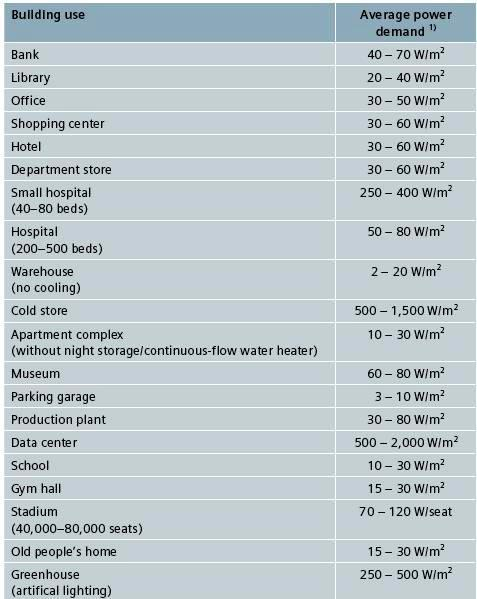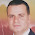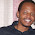Electrical Load Estimation – Part Two

In the previous topic " Electrical Load Estimation – Part One " , I explain the definitions of the most important terms in electrical load estimation and provides download links for factors used in the electrical load estimation.

Also, I listed the (5) methods of electrical load estimations which were:

A- Preliminary load calculation which divide to:

1. Space by space (functional area method),
2. Building Area method.
3. Area method.

Today, I will explain these methods for electrical load estimations.

Note:

I will explain the (3) preliminary load estimation methods only in this course, and the two other methods, NEC load calculations and Final load calculations will be explained later in course "
EE-3: Basic Electrical design course – Level II " , because these two methods are applied in the final stages of design.

Difference between preliminary and final load estimate

 S/N Preliminary load calculations Final load calculations 1 Units of Loads will be in (W/ft2) watts per square foot or/and (VA/ft2) volt-amperes per square foot Units of Loads will be in KW (kilo-watt), or/and KVA (kilo-volt-ampere), or/and HP (horse power) 2 units are used interchangeably because unity power factor is assumed Units can’t used interchangeably. So, Hp will be converted to kVA; and kVA may be multiplied by the estimated power factor to obtain kW if required 3 Unity power factor is assumed Different values of power factors according to load types. 4 Demand and load factors values will be selected from tables based on the designer estimation and they will be Used to calculate the transformer and service size. Demand and load factors values  are Real values that will document and reflect the number, the type, the duty rating (continuous, intermittent, periodic, short time, and varying), and the wattage or volt-ampere rating of equipment supplied by a common source of power, and the diversity of operation of equipment served by the common source. 5 The connected load will be estimated based on area or population Actual demand load will be calculated based on summation of individual building connected loads modified by suitable demand and diversity factors 6 Easy and Fast calculations economical, cost effective calculations insuring that items of equipment and materials are adequate to serve existing, new, and future load demands

Preliminary load calculations will be divided to:
1. Space by space (functional area method),
2. Building Area method,
3. Area method.

Note:

A particular design may use one Preliminary load estimate method or a combination from two or even the three methods.

First: Space-by-Space Method (functional area method)

In the Space-by-Space Method, the building will be divided into different space based on its function like offices, conference halls, corridors and lobbies, shops, parking areas, workshops and etc. The Load density in (W/ft2) or/and (VA/ft2) is prescribed for these different spaces, these load densities in addition to spaces area will be used to estimate the preliminary electrical load of this building as described in below.

Usage conditions of Space-by-Space Method

• The Space-by-Space Method is used only for individual spaces in the building.
• The Space-by-Space Method may be used for any building or portion of a building.

Area Measurement in space by space method

The square footage is measured from the outside surface of exterior walls to the centerline of walls between interior partitions of the spaces. The sum of the Gross Interior Area equals the total Gross Area of the building.

Method of estimation by using Space-by-Space Method

In this method, we have two cases as follows:

• First case: availability of grouped load density (i.e. one value covering all lighting, general power and power loads) in (W/ft2) or/and (VA/ft2) for each space.
• Second case: availability of individual load density (i.e. individual values for lighting, general power and power loads) in (W/ft2) or/and (VA/ft2) for each space.

First case

Method of estimation by using Space-by-Space Method will be as follows:
1- Divide the building into different space based on its function (for example, office, storage, mechanical, and corridor).

2- Calculate the gross interior area of each space.

3- Determine the grouped load density for each space (from tables).

4- Multiply each space gross interior area by its grouped load density to get the estimated electrical load for this space.

5- Sum all the estimated electrical loads for all spaces to get the total preliminary electrical load for the whole building.

6- Multiply the total preliminary electrical load for the whole building by the power factor value (= 0.8) and a suitable load factor (for future extensions and losses compensations) to get the main service size.

Example# 1:

For a high-rise office building with shopping arcade, the power supply has to be planned for a 10-story building (12 floors) with a floor area of approx. 25 m x 60 m.

There is a car park for customers in front of the building, the access way to the parking garage (levels -1 to -3 for employees) and a fountain (80 m x 20 m). (Fig. 1)

Real floor area approx. 1350 m2 (14 levels + technical equipment on roof level).

Floor heights of levels 00 to +10: 4 m, levels -1 to -3: 3 m
Calculate main electrical service size for this building.Fig (1)

The solution:

Consider first case: availability of grouped load density for each space. Hence, calculations will be as follows:

1- The building will be divided to the following spaces:

• Parking garage / utilities areas (incl. roof area)
• Shopping center / bank
• Offices

2- Calculate The gross areas of each space will be as follows:

Parking garage / utilities areas (incl. roof area) Basement levels -1 to -3 with 1,350 m2 each + utilities areas ca. 210 m2 (areas between utilities hubs),
Area = (3 x 1,350 m2 + 210 m2) = 4260 m2

Shopping center / bank Ground level 00 with 1,350 m2
Area = 1 x 1,350 m2 = 1350 m2

Offices Levels +1 to +10 with 1,350 m2 each
Area = 10 x 1,350 m2 = 13500 m2Table# 1

3- Determine the grouped load density for each space (from table#1) as follows:

Parking garage / utilities areas (incl. roof area)
assumed average power demand: 10 W/m2
Shopping center / bank
assumed average power demand: 60 W/m2
Offices
assumed average power demand: 50 W/m2

4- Multiply each space gross interior area by its grouped load density to get the estimated electrical load for this space as follows:

Parking garage / utilities areas (incl. roof area)
Estimated electrical load = (3 x 1,350 m2 + 210 m2) x 10 W/m2 = 42,600 W
Shopping center / bank
Estimated electrical load = 1 x 1,350 m2 x 120 W/m2 = 162,000 W

Offices
Estimated electrical load = 10 x 1,350 m2 x 110 W/m2 = 1,485,000 W

5- Sum all the estimated electrical loads for all spaces to get the total preliminary electrical load for the whole building.

Total preliminary electrical load for the whole building = 42,600 + 162,000 + 1,485,000 = 1,690 kW

6- Required transformer output

The established total power demand determines the required transformer output. The determination is based on a cos phi = 0.85 and a transformer load level of 70%.
Transformer output rating = 1,690 kW / (0.7 x 0.85) = 2,840 kVA

Second Case

Method of estimation by using Space-by-Space Method will be as follows:

1- Divide the building into different space based on its function (for example, office, storage, mechanical, and corridor).

2- Calculate the gross interior area of each space.

3- Calculate the estimated lighting, small appliance and power loads for each space as follows:

• Determine the type of light source for each space.
• Determine the Lighting load density in (W/ft2) or/and (VA/ft2) for this type of light source.
• Consider requirements for supplementary lighting (for example, floodlighting, security lighting, and special task lighting).
• Multiply the gross interior area of the space by the assigned Lighting load density to get the estimated lighting electrical load for this space.

• Small appliance loads shall include those served by general purpose receptacles. In general, the dividing of areas by function for estimating lighting loads will serve for estimating small appliance loads.
• Determine the Small Appliance load density in (W/ft2) or/and (VA/ft2) for this space.
• Consider requirements for the extent of using small appliances by the occupants of this space (for example, Mechanical spaces in building storage areas and similar spaces in which outlets are provided but infrequently used are usually neglected in computing loads, except for special cases).
• Multiply the gross interior area of the space by the assigned Small Appliance load density to get the estimated Small Appliance electrical load for this space.

• Electric power loads shall include all loads other than lighting loads and those served by general purpose receptacles and comprise the environmental system electric power requirements and the facility occupancy equipment electric power requirements.

HVAC Equipment
• Use the same method for small appliance loads

Lifts and Escalators, Pumps and other loads

• These loads are related to the whole building and not related to the divided spaces. So, The Space-by-Space Method cann’t estimate the required ratings for these loads and we must use the building method for this purpose.

4- After computing the preliminary electrical loads for each space, we must sum all of them to get the total preliminary electrical load for the whole building.

Example# 2 :

Consider the same building in example# 1 to be solved if individual load densities are known for each load type as follows:

 Space Load Load Density (W/M2) Parking Garage / Utilities Areas (Incl. Roof Area) Lighting 7 Small Appliance 3 Power Load Inc. in building power Shopping Center / Bank Lighting 28 Small Appliance 20 Power Load Inc. in building power Offices Lighting 28 Small Appliance 20 Power Load Inc. in building power Building Power Load HVAC - Pumps - Elevators - Others 60

Calculate main electrical service size for this building.

The solution:
1- The following table summarizes the calculations steps.

 Space Area Load Load  Density Estimated  Load Estimated  Electrical  Load For  Space Parking Garage /  Utilities Areas  (Incl. Roof Area) 4,260 Lighting 7 29,820 42,600 VA 4,260 Small Appliance 3 12,780 4,260 Power Load 0 0 Shopping Center /  Bank 1,350 Lighting 28 37,800 64,800 VA 1,350 Small  Appliance 20 27,000 1,350 Power Load 0 Offices 13,500 Lighting 28 378,000 648,000 VA 13,500 Small  Appliance 20 270,000 13,500 Power Load 0 0 Building Power  Load 14,850 HVAC – Pumps – Elevators –  Others 60 891,000 891,000 VA Total Estimated Electrical Load 1,646,400 VA Application Of Cos Phi = 0.85 1,936,941 VA Application Of Load Level = 70% 2,767,059 VA Transformer Output Rating 2,767 KVA

In the next topic, I will explain Other methods for preliminary electrical load calculations. So, please keep following.

1.the best thing in your lessons is the examples , keep going and we with you .

1.thanks, medo88

2.شكر الله لك مازلت أستفيد منك دائما وأكمل مشروعك التعليمي

3.This comment has been removed by the author.

4.Building Power
Area14,850m2-how did we arrive at this?

1.Area14,850m2 = shooping Center + officies = 1350 + 13500 = 14850 m2
parking garage and utilites area are not served for building power load (HVAC –Pumps –Elevators – Others)

2.thanks,good work that you are doing.

5.Example #1
Shopping center / bank
assumed average power demand: 60 W/m2
Offices
assumed average power demand: 50 W/m2

but in calculation you used 110w/m2 and 120 w/m2 whats the logic behind this

6.correct me if am wrong, but I think in the first example when calculating for transformer output, we should multiply the total power 1,690KW by 0.7 instead of dividing by the same.

1.Load level 70% means that the load of the transformer must be equal or less than 70% of the transformer rating . so, 1,690 KW must equal 70% of the transformer rating. how much is the transformer rating? of course must be more than the load 1,690 KW and therefore, the load is divided by( the 70% multiplied by the P.F=0.8.)

7.hi Ali,
Could you inform us fr average demand we can refer to IEC standard number ?

8.Keep up with good work.